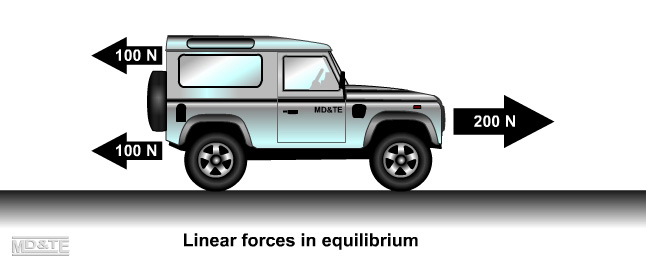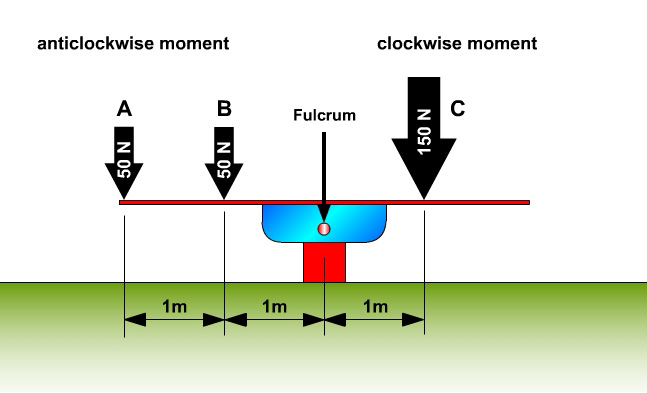### Equilibrium is balance.

For an object to be in equilibrium:

• the sum of the linear forces acting on the object in any direction must = 0
• the sum of the moments at any point must = 0

1. Equilibrium: the sum of the linear forces acting on the object in any direction must = 0• The forces acting toward the left = 200N
• The force acting toward the right = 200N

The forces acting toward the left cancel out the forces action to the right so the vehicle is in equilibrium.

2. Equilibrium: the sum of the moments at any point must = 0

An object is in equilibrium, i.e. it is balanced and not turning or moving when the sum of the clockwise moments about a point is equal to the sum of the anticlockwise moments about the point.The see-saw is balanced because the clockwise moment is equal to the sum of the anticlockwise moments, i.e.

Moment = Force x Distance

Moment at A = 50N x 2m = 100Nm anticlockwise moment

Moment at B = 50N x 1m = 50Nm anticlockwise moment

Anticlockwise moment at A + B = 150Nm

Moment at C = 150N x 1m = 150Nm clockwise moment

The see-saw is balanced because the clockwise moment is equal to the sum of the anticlockwise moments.